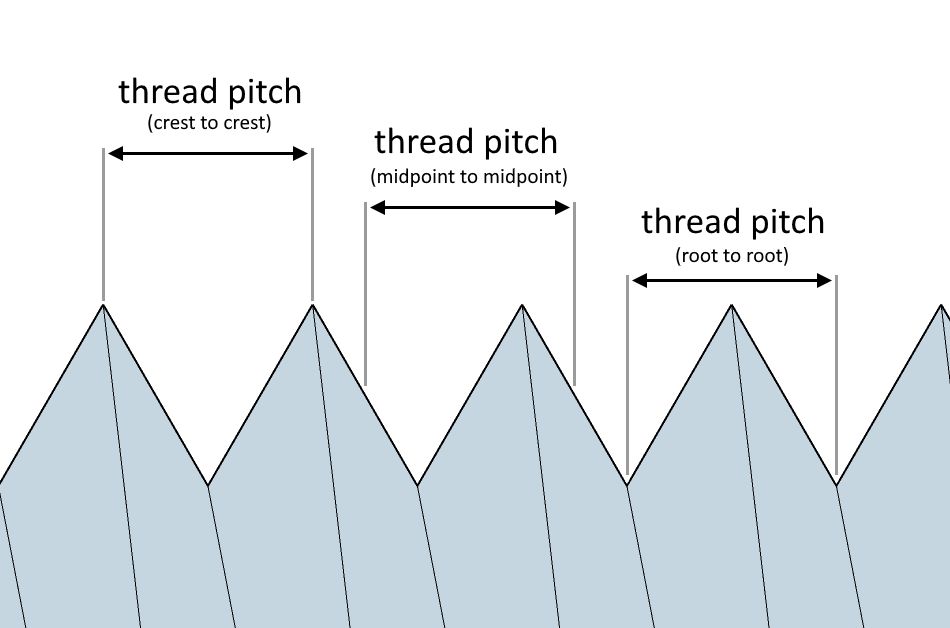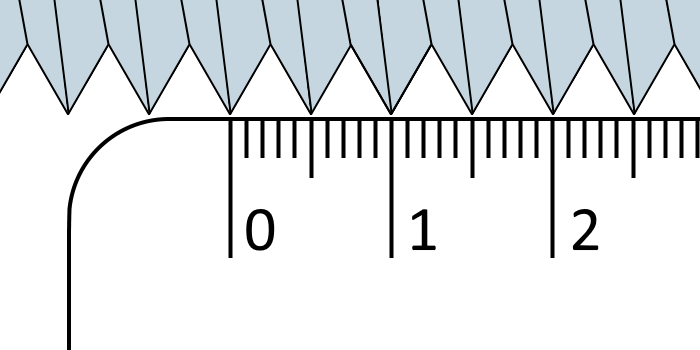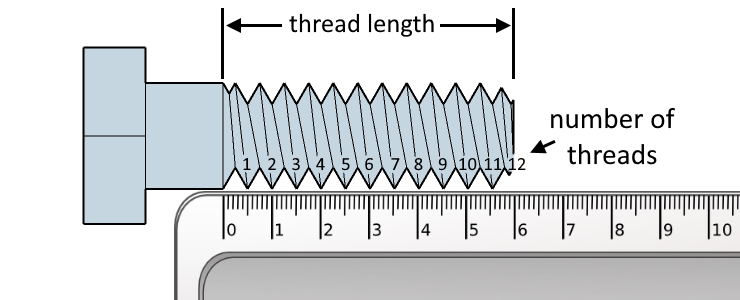# Thread Pitch Calculator

Created by Kenneth Alambra
Reviewed by Wojciech Sas, PhD candidate and Jack Bowater
Last updated: Sep 25, 2022

This thread pitch calculator will help you find the pitch of your threaded fastener. This tool also works as a TPI, or threads per inch, calculator and a number of threads calculator. In this calculator, you will learn:

• What thread pitch is;
• How to measure thread pitch using a ruler;
• How to calculate thread pitch using the thread pitch formula; and
• How to find thread pitch given threads per inch.

Keep on reading to start learning 🙂!

## What is thread pitch?

Thread pitch, or pitch, in short, is the distance between the same point on two adjacent threads. Thread pitch can be the length between two crests or two roots of a thread.

As long as we measure two identical points on two threads next to each other, we are measuring the thread pitch. Thread pitch also tells us how wide a threaded fastener's thread is. You can refer to the illustration below to see what a thread pitch can be:🔎 Are you looking for fastening materials that don't have threads? Using rivets is one of your options. Find the correct size of rivets to use using our rivet size calculator.

## How to measure thread pitch with a ruler

The easiest way to measure thread pitch with a ruler (or with a caliper for a more precise measurement) is by measuring the distance between two crests. Match a thread's crest to your ruler's zero-mark and read the length to the next crest, as shown in the diagram below:You can measure the thread pitch using millimeters or inches. If you notice that all the threads sit on the millimeters scale like in the illustration above (a 5-millimeter thread pitch), you must have a metric thread. Learn more about metric threads with our thread calculator.

On the other hand, if a certain number of threads fit exactly within an inch, you must have an inch thread.

However, there are times that threads don't match either the millimeter or inch scale. That's when knowing how to calculate thread pitch comes in handy. Learn how in the next section of this text.

## How to calculate thread pitch

It is very easy to calculate the thread pitch of any thread. First, measure the entire length of the thread you want to check. Then, count the threads along that length. This is the same as knowing how many times this fastener has to rotate to engage all its threads. Check the illustration below to understand better what to measure and count:After measuring these values, we can now use the thread pitch formula:

P = L / n,

where:

• P - Thread pitch;
• L - Thread length; and
• n - Total number of threads along the thread length.

## How to find thread pitch given threads per inch

If we measure the thread length in inches and divide the total number of threads by the thread length in inches, we get the threads per inch (TPI) value of a given thread. We can also express this statement in equation form, as shown below:

TPI = n / L

Taking a closer look at this equation, we can see that TPI is simply the reciprocal of the thread pitch. With that said, we can find thread pitch by taking the reciprocal of TPI as shown in this equation:

P = 1 / TPI

Now that we know how to find thread pitch using the thread pitch formula and the TPI of a thread, why don't we learn how to use the thread pitch calculator? 🙂

## How to use the thread pitch calculator

Here are the steps to follow to use our thread pitch calculator:

1. Enter the length of the threaded part of your threaded fastener.
2. Input the total number of threads along the length you entered.
3. If you know your thread's threads per inch value instead, you can skip Steps 1 and 2 and type in the threads per inch value directly.

After completing the steps above, you'll instantly find your thread's pitch! 🔩

You can also use our calculator to find the number of threads on your threaded fastener. Just enter the thread length and a value for either TPI or thread pitch, and you'll get the total number of threads.

✅ Craving for more knowledge about thread pitches? Check out our pitch diameter calculator to learn more! 🙂 On the other hand, if you're fastening a round flange or plate, you might find our bolt circle calculator helpful in determining the placement of your bolts.

## FAQ

### What is the thread pitch of a 2-inch long bolt that has a total of 48 threads?

To find the thread pitch of a bolt that is 2 inches long with 48 threads:

1. Divide 2 inches by 48 threads.
2. The result is a 0.04167 inch thread pitch.

To get the thread pitch in millimeters:

1. Multiply 2 inches by 25.4 millimeters per inch to get 50.8 mm.
2. Divide 50.8 mm by 48 threads to obtain a thread pitch of 1.0583 mm.

### What is metric thread pitch?

Metric thread pitch is the distance between two threads of an ISO metric thread, and we usually express metric thread pitches in millimeters. In a metric thread callout (or the label of a threaded fastener), the metric thread pitch is the number after the × symbol. For example, an M10 × 1.25 bolt has a 1.25-millimeter thread pitch.

### What is a thread pitch gauge used for?

Besides rulers and calipers, we can also use thread pitch gauges to find the thread pitch of a threaded fastener. Thread pitch gauges come in sets of toothed shims that we match with a thread to find the thread's pitch. Each toothed shims has a particular thread pitch that we can also see etched on its face.

### How to figure out thread pitch?

For a M36 × 4 metric thread callout, the second number 4 represents the threaded fastener's 4 millimeters thread pitch. On the other hand, for a 3/4" - 10 UNC unified coarse thread callout, the value 10 is the threads per inch value of the thread. By dividing 1 inch by this value of 10 threads per inch, we get a value of 0.1-inch thread pitch.

Kenneth Alambra
in
Total number of threads (n)
Threads per inch (TPI)
in
People also viewed…

### Alien civilization

The alien civilization calculator explores the existence of extraterrestrial civilizations by comparing two models: the Drake equation and the Astrobiological Copernican Limits👽

### Coffee kick

A long night of studying? Or maybe you're on a deadline? The coffee kick calculator will tell you when and how much caffeine you need to stay alert after not sleeping enough 😀☕ Check out the graph below!

### Concrete

Concrete calculator - slab evaluate the amount of concrete and cement needed for a slab block.

### Square footage

Square footage calculator turns length and width into an area and converts to/from multiple units.